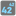• # Why doesn't this work?

## Question related to missionIndex Power

Problem: You are given an array with positive numbers and a number N. You should find the N-th power of the element in the array with the index N. If N is outside of the array, then return -1. Don't forget that the first element has the index 0.

Let's look at a few examples: - array = [1, 2, 3, 4] and N = 2, then the result is 32 == 9; - array = [1, 2, 3] and N = 3, but N is outside of the array, so the result is -1.

Can anyone help me figure out why this doesn't work?

def index_power(array, n):

```for i in array:
if array.index(i) == n:
return i**n
elif n > len(array)-1:
return -1
```4